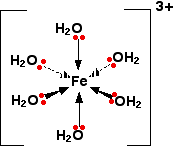# Question #d5ed4

Nov 8, 2017

You can minimise it by lowering the pH.

#### Explanation:

Iron(III) forms a hydrated complex:As electron density is drawn towards the central metal ion this tends to remove electron density away from the O - H bond causing the hydrogen atoms to have a greater $\delta +$ charge on them.

In aqueous conditions this means that they will be more strongly attracted to the water molecules so tend to undergo hydrolysis:

$\textsf{{\left[F e {\left({H}_{2} O\right)}_{6}\right]}^{3 +} + {H}_{2} O r i g h t \le f t h a r p \infty n s {\left[F e {\left({H}_{2} O\right)}_{5} O H\right]}^{2 +} + {H}_{3} {O}^{+}}$

$\textsf{\textcolor{p u r p \le}{\text{ ""pale lilac")" " " " " " " " " " " """color(orange)("orange}}}$

This means a solution of an iron(III) salt is quite acidic.

If you apply Le Chatelier's Principle you can see that raising the hydronium ion concentration should drive the position of equilibrium to the left, thus suppressing the hydrolysis.

Typically a small amount of conc. nitric acid may be used.